### Long Transmission Line

In the last article we discussed short and medium length lines. Now is the time for long transmission lines. For long transmission lines if we apply the formula for medium length line we will get large error. The error becomes larger with longer transmission lines and the method is useless.  So for long lines it becomes important to represent accurately. Hence the long transmission lines are represented by distributed parameters.

Long Line Model

For medium length line we lumped the line impedance at one place. This line impedance which is actually distributed throughout the length of line will be represented here as distributed.  The capacitive current between the line conductors flows throughout the length of line. Which necessitates for distributing the line to line capacitance throughout the length of the line.  But for a balanced three phase system we  analyse per phase analysis. Which necessitates for consideration of phase to neutral capacitance, Both line inductance and capacitance for several configurations are illustrated in previous articles. The equivalent long line representation is shown in Fig-A.

In the diagram the distributed capacitance also automatically requires that the line impedance also distributed. It should be clear from the above figure that in general throughout the length of the line the voltage and current values may vary.

What is the voltage and current at a distance x from the receiving end. See Fig-B Let the voltage and current at a distance x from receiving end are V(x) and I(x) respectively. It should be noted that V(x) and I(x) are phasors.

Let  Z= √(Z/Y)
and   γ =√(ZY)

As Z and Y are complex numbers so in general Z and γ are complex numbers. Z is called the characteristics impedance and γ is the propagation constant. Z is commonly called surge impedance in power sector.

Formula for calculation of Z and Y are already discussed in previous articles.
Z is the series impedance per unit length ( impedance per kilometer or meter)
Y is the shunt Admittance per unit length

Using differential calculus and solving the resulting differential equations it can be shown that,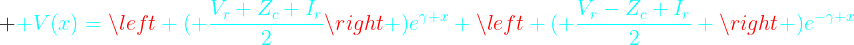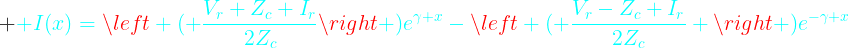The above formula can be rearranged and written in several ways. Rearranging the terms and using the formulas of complex hyperbolic, the above formulas for V(x) and I(x) can be written in the form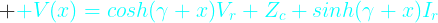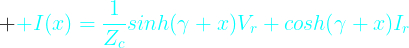To find the voltage Vs and current Is at the sending end, we just have to put l (length of line) in place of x  in the above formulas. So at the sending end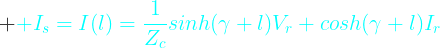If you compare the above equations relating sending and receiving end voltage and current with the corresponding equations discussed in last article for medium length lines then we can easily find ABCD parameters. These are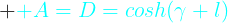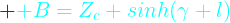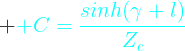You can argue that the long transmission line can also be represented by a nominal  ∏ circuit. Yes a long line can be represented by an equivalent ∏ circuit. It should be remembered that in case of medium length line it is called Nominal ∏. Here it is called equivalent  ∏. This equivalent ∏ is only a convient representation of the actual long line analysis. But Nominal ∏ is an approximation for medium lines. For load flow study and other system studies this equivalent representation is very helpful without sacrificing any accuracy. In Fig-C is shown the equivalent ∏ representation of any line of length l according to long line theory.

It is left as an exercise for you to find Z' and Y' by comparing with Medium length line.

In all the above formulas we used two parameters Zand  γ. These two parameters are very important. Of course these two parameters are derived from our transmission line parameters Z and Y. The characteristics of the long line depends upon these two  parameters.

As already said Zc  and γ  are complex numbers.

Let us consider a case when the load impedance is just equal to the characteristics impedance Zc   then, the receiving end voltage  Vr  =IZc

substituting in above equations we get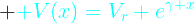and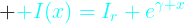let, γ  = α+j β

Dividing V(x) by I(x) we get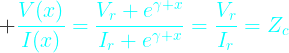From the above equation it is easy to interpret that the impedance as seen at any point of the line is the same as the load impedance that is Z, the characteristics impedance. Moreover from the above equations of  V(x) and I(x) it is clear that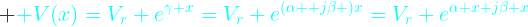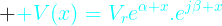The magnitude of voltage is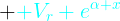Clearly the magnitude of the voltage increases with x. But our x increases from receiving end to sending end. So the voltage increases exponentially from receiving end to sending end.

At the sending end the voltage is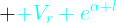The other term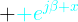is the phasor and only provides phase shift between the voltages at receiving end and at a point x distance from the receiving end. Similar argument can be made for equation of current I(x).

In the above formula of Zc and γ  we put Z(series impedance) and Y(shunt admittance). (recall that Z and Y are the values per unit line length)

Loss Less Line

What will happen for a loss less line. For loss less line R=0 and G=0.
So our above equations reduces to

Observe that for loss less line, Zc becomes a pure resistance. We also know that γ  = α+j β,  for this loss less situation we get

Now our previous voltage and current equations for surge impedance loading reduces to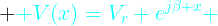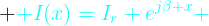As in case of lossy line here also at any distance x from receiving end the ratio of voltage and current is always same that is Zc, the surge impedance of the line. Using the complex algebra you are sure that the magnitude of V(x) is Vr and I(x) is Ir. Which means that for loss less line the voltage and current at any distance x from the receiving end is same. It also implies voltage at sending end is same as voltage at receiving end which is same as voltage at any intermediate point. So Vs = V(x) = Vr. At Surge Impedance Loading the reactive power generated by the line capacitance is equal to the reactive power absorbed by the line inductance for every unit length of line. In Power industry it is said that the voltage profile is flat. So we conclude that for a lossless line the voltage magnitude is same throughout the length of line. As in case of lossy line the term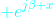is the phasor responsible for phase shift. It is simply giving phase shift to the voltage wave along the length of line. The phase angle between sending and receiving end voltage is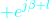. It is clear that if the distance between the sending and receiving end is more then the phase difference between the voltage phasors at both the ends of the line will be more.

In previous articles we already discussed that in case of transmission line how and when we can ignore the line resistance R and  leakage  conductance G. At least for rough estimate of the load carrying capability of transmission line we can presume it lossless. The surge impedance loading is the ideal loading of the line, which is desired keeping in view of the optimised (flat for lossless ideal case) voltage profile of the line.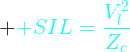Where

It should be recalled that Zc is pure resistance for lossless line.

Vr and Vl are the receiving end phase and line voltage respectively.

Approximate SIL for few nominal voltages
• 132/138 kV  -    50 MW
• 230 kV         -  150 MW
• 345 kV         -  400 MW
• 400 kV         -  500 MW
• 500 kV         -  900 MW
• 765 kV         -  2090 MW

Above values of SIL is true for both 50 Hz and 60 Hz systems.

System planners usually use line loadabilty curve for deciding loading capability of the line. See Fig-D. The relationship between SIL and length in km shown in Fig-D is almost same for all voltage levels.

What is the capacity of the transmission line or how much power it can carry. The power that a transmission line can carry are based on three factors. These are
• Thermal Limit
• Voltage Drop Limit
• Stability Limit
Due to the current flow heat is generated in the line and the line length changes which gives rise to more sag.  Sometimes heating of the line is enough that, later cooling of the line due to less load or environment factors does not make the line regain its actual length. The sagging become permanent. Due to this the minimum clearance of the line to ground decreases which may violates the standard set by the local authority. Also if the load is very high the conductor may be damaged due to excessive heat. All transmission lines has thermal limits. But the thing is that only short lines can approach this limit. Voltage drop and stability limits situation usually do not arise here due to short length. Lines less than 80 km length falls in this category.

For medium length line the loading is mainly limited by allowable voltage drop (usually between 5 to 10 % as set in grid standard). For medium length line the steady state stability limit situation usually does not arises due to lesser length(discussed below). But the length is enough so that the medium length line can encounter the voltage drop limit before reaching thermal limit. By reactive compensation the voltage drop limit can be increased. Lines exceeding 80 km and less than 250 km long belong to this category.

In both lossy and lossless lines it is clear that phase difference between sending and receiving end voltage arises. This angle is called power angle. The power that flows from sending to receiving end depends upon this angle and the magnitude of Vs and Vr. (The magnitude of a phasor V is represented as |V| )

The simplified formula for this power (P) transmitted is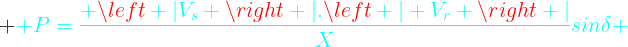X is the equivalent series reactance(ignoring resistance) of the line.

To increase the power to be transmitted, this power angle δ may increase up to 90 degrees. Increasing slightly further, the line becomes unstable and lose synchronism. It is a good practice to operate the lines with sending and receiving ends phase differnce angle (power angle) less than 30 degrees.  Doing so, if in emergency load generation disbalance in adjacent areas occurse this line can take more load by increasing this power angle, so avoiding instability. So operating below 30 degrees we keep more than 60 degree angle margin. From the above formula you can say that if X is made smaller and smaller for any fixed small δ ( small sin δ  imply small δ)  then more power can be transmitted. Hence it is clear that small line reactance X is desired. This small reactance which cannot be made arbitrarily small by line design, definitely limit the power transmission in line.  This loading limit for transmission line is quite less than thermal limit.  So the long lines cannot approach thermal limit, before that other limits come to action. This small power angle corresponds to quite lesser load carrying capacity in comparison to thermal limit. Effort is made by power companies to push the limit towards thermal limit by employing reactive compensation so reducing effective series X further.ahmed mohamed abdelfatah said...

can any body give me the comparison between the
long , short & medium transmission line
the effect of changing power factor on the percentage error in each moduleAnonymous said...

what is the INPUT IMPEDANCE OF A TRANSMISSION LINE?